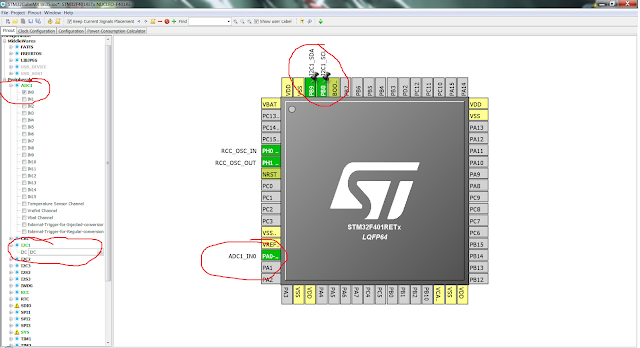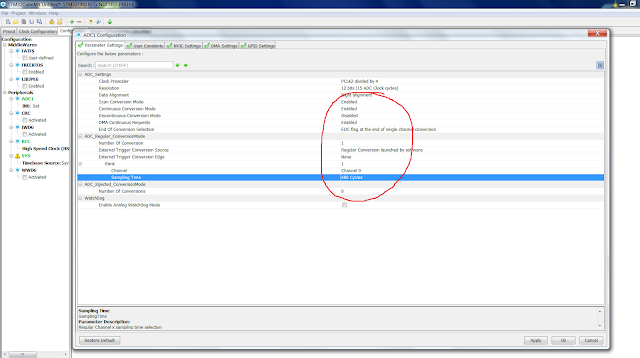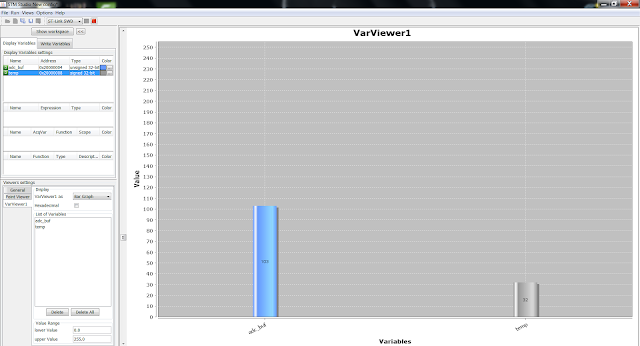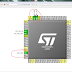LM35 is an integrated-circuit temperature device with an output voltage linearly proportional to the centigrade temperature. As LM35 is not calibrated in Kelvin, user does not require to subtract large constant voltage from the output to obtain convenient centigrade scaling.

## Features of LM35

• Calibrate directly in celcius
• 0.5°C Ensured Accuracy ( at 25°C)
• Rated for full -55°C to 150°C Range
• Suitable for Remote Applications
• Operates from 4V to 30V
• Less than 60μA current drain
• Low Self-Heating, 0.08°C in Still Air
• Non-Linearity Only ±¼°C Typical
• Low-Impedance Output, 0.1 Ω for 1-mA Load

## Applications

• Power Supplies
• Battery Management
• HVAC
• Appliances

We will use ADC to read values from LM35, And display it on the lcd. Lcd is connected via I2C using PCF8574. If you don't know how to use DMA with ADC, check out this Tutorial. To know more about connecting LCD via I2C, go HERE.

Vcc is given to pin1, and pin3 is connected to the ground. Pin2 will be the input to the ADC pin of the microcontroller.

## CubeMx Setup

Create new project in CubeMx and select desired ADC channel.ADC1 channel 0 is selected and I2C1 is selected
Go to ADC1 config in configuration tab and make sure your settings are as followsScan Conversion- Enabled Continuous conv- Enabled DMA request - Emabled Sampling time - Highest(480 cycles)

Go to DMA setting and select the following

That's it for CubeMx setup. Generate the project and open it

## Some Insight into the Code

create variables:-

``````uint32_t adc_buf;
int temp;
``````

uint32_t adc_buf is the ADC buffer, where values from LM35 will be stored.
int temp is the variable to store the temperature.

write a callback function:-

``````void HAL_ADC_ConvCpltCallback(ADC_HandleTypeDef* hadc)
{
temp = adc_buf*.322; // convert value from adc buffer to temperature in Centigrade
}
``````

Write functions for LCD
``````
/* Send command function */
void lcd_send_cmd (char cmd)
{
char data_u, data_l;
uint8_t data_t;
data_u = cmd&0xf0;
data_l = (cmd<<4)&0xf0;
data_t = data_u|0x04;  //en=1, rs=0
data_t = data_u;  //en=0, rs=0
data_t = data_l|0x04;  //en=1, rs=0
data_t = data_l;  //en=0, rs=0
HAL_I2C_Master_Transmit (&hi2c1, 0x4E,(uint8_t *) data_t, 4, 100);
}

/* send data function */
void lcd_send_data (char data)
{
char data_u, data_l;
uint8_t data_t;
data_u = data&0xf0;
data_l = (data<<4)&0xf0;
data_t = data_u|0x05;  //en=1, rs=0
data_t = data_u|0x01;  //en=0, rs=0
data_t = data_l|0x05;  //en=1, rs=0
data_t = data_l|0x01;  //en=0, rs=0
HAL_I2C_Master_Transmit (&hi2c1, 0x4E,(uint8_t *) data_t, 4, 100);
}

/* lcd initialize function */
void lcd_init (void)
{
lcd_send_cmd (0x02);
lcd_send_cmd (0x28);
lcd_send_cmd (0x0c);
lcd_send_cmd (0x80);
}

/* send the whole string at once */
void lcd_send_string (char *str)
{
while (*str) lcd_send_data (*str++);
}
``````

Initialize LCD in the main function
``````lcd_init ();
``````

``HAL_ADC_Start_DMA (&hadc1, &adc_buf, 1);``

Convert the temp (integer) to temperature (character)

``````char temperature;

sprintf (temperature, "%d", temp);
``````

send the temperature to the LCD

``````lcd_send_string ("Temp= ");

lcd_send_string (temperature);

lcd_send_string ("C");

lcd_send_cmd (0x86);``````

## RESULT

The following is the output in STMStudio. Variables observed are adc_buf and temp.adc_buf value is 103 and temp is 32
As you can see below the LCD showing temperature of the surrounding.

LM35 Temperature sensor using STM32Reviewed by arun singh on September 07, 2017 Rating: 5